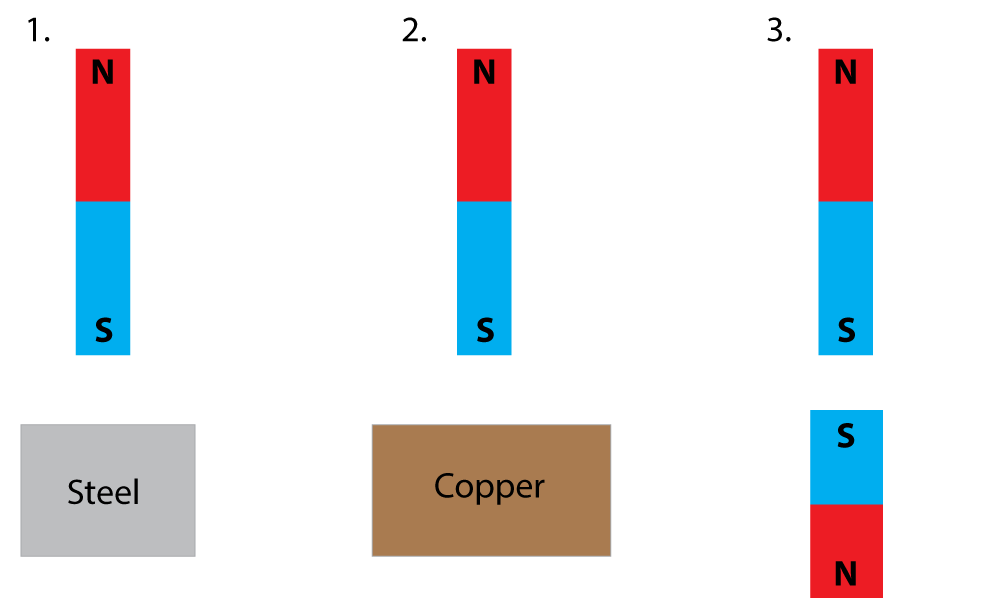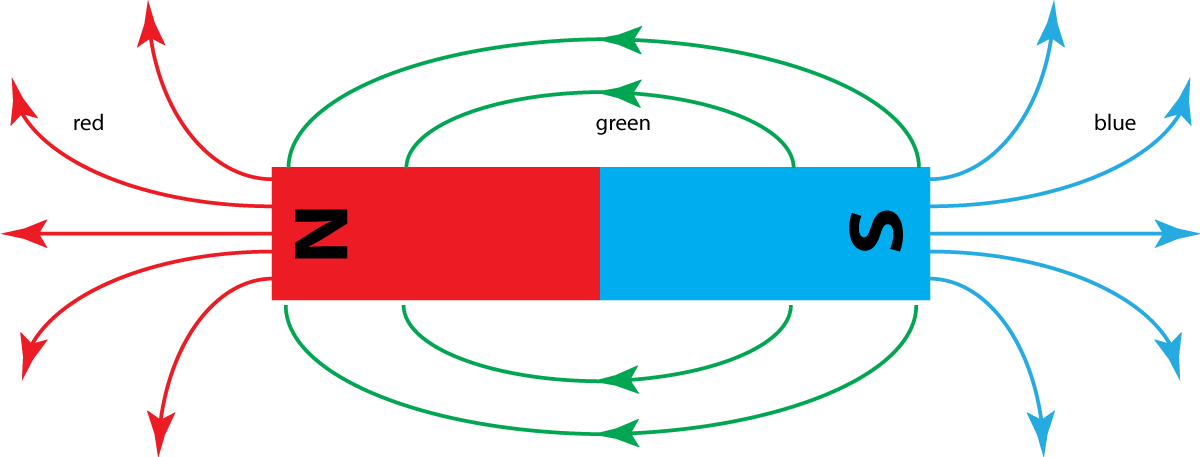10 minutes maximum! Can you do it in 5?

1. Which of these is a magnetic material?

• A. Iron
• B. Glass
• C. Plastic
• D. Copper

2. The table shows how 2 different poles of magnets attract or repel. Which row is correct?

 North and North pole North and South pole A attract attract B attract repel C repel attract D repel repel

3. Here are three statements about magnets:

• I. Magnets can be made using any metal.
• II. Magnets produce forces on other magnets.
• III. Magnetic field lines are drawn with arrows pointing from north to south.

Which of these statments are true?

• A. II only.
• B. I and II.
• C. II and III.
• D. I and III.

4. What do magnetic field lines show?

• A. The direction the south pole of a compass would point.
• B. The direction the north pole of a compass would point.
• C. The direction of force on a positive charge.
• D. The direction of force on a negative charge.

5. Some substances can be magnetised, and hold on to their magnetism for many many years.

This kind of magnet is called....

• A. a permanent magnet.
• B. a solid magnet.
• C. a soft magnet.
• D. an induced magnet.

6. Magnetic compasses point North. This shows that..

• A. the Earth has a magnetic field.
• B. the Earth is spinning.
• C. the Sun has a magnetic field.
• D. the Earth has a electric field.
7. An experiment is performed on 3 metals as shown below. A bar magnet is used to try to pick up a steel block, a copper block and another small bar magnet.Which row in this results table correctly shows the 3 results?

 1. Steel 2. Copper 3. South Pole A attract no effect attract B no effect attract repel C attract no effect repel D attract attract repel

8. In test 3 above, it was noticed that the distance between the 2 magnets made a big difference. Which of the statements below best describes this conclusion?

• A. If the distance between magnets is increased, the force gets stronger.
• B. If the distance between magnets is increased, the force gets weaker.
• C. If the force is increased, the distance gets bigger.
• D. If the force is increased, the distance gets smaller.

9. At which point on this diagram (labelled A to D) is the magnetic field strongest?10. Maggie has a go at drawing the field lines around a bar magnet. However she has got some of the arrow directions the wrong way round.Which colour arrows are pointing the wrong way?